This mathematics ClipArt gallery offers 100 illustrations of United States coins that add up to values from one cent to one dollar.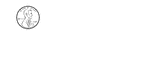### 1 Cent

Groups of change with totals from 1 to 100 cents using the least amount of coins.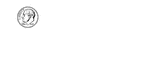### 10 Cents

Groups of change with totals from 1 to 100 cents using the least amount of coins.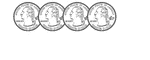### 100 Cents

Groups of change with totals from 1 to 100 cents using the least amount of coins.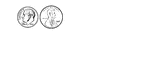### 11 Cents

Groups of change with totals from 1 to 100 cents using the least amount of coins.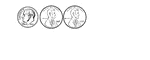### 12 Cents

Groups of change with totals from 1 to 100 cents using the least amount of coins.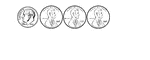### 13 Cents

Groups of change with totals from 1 to 100 cents using the least amount of coins.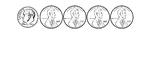### 14 Cents

Groups of change with totals from 1 to 100 cents using the least amount of coins.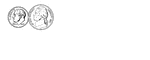### 15 Cents

Groups of change with totals from 1 to 100 cents using the least amount of coins.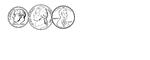### 16 Cents

Groups of change with totals from 1 to 100 cents using the least amount of coins.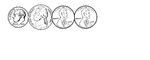### 17 Cents

Groups of change with totals from 1 to 100 cents using the least amount of coins.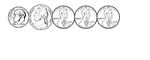### 18 Cents

Groups of change with totals from 1 to 100 cents using the least amount of coins.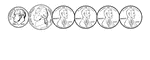### 19 Cents

Groups of change with totals from 1 to 100 cents using the least amount of coins.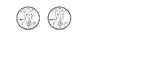### 2 Cents

Groups of change with totals from 1 to 100 cents using the least amount of coins.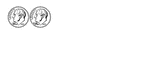### 20 Cents

Groups of change with totals from 1 to 100 cents using the least amount of coins.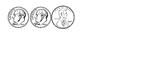### 21 Cents

Groups of change with totals from 1 to 100 cents using the least amount of coins.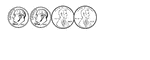### 22 Cents

Groups of change with totals from 1 to 100 cents using the least amount of coins.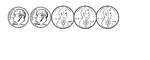### 23 Cents

Groups of change with totals from 1 to 100 cents using the least amount of coins.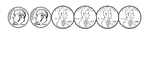### 24 Cents

Groups of change with totals from 1 to 100 cents using the least amount of coins.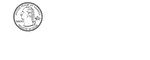### 25 Cents

Groups of change with totals from 1 to 100 cents using the least amount of coins.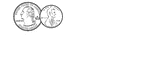### 26 Cents

Groups of change with totals from 1 to 100 cents using the least amount of coins.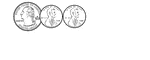### 27 Cents

Groups of change with totals from 1 to 100 cents using the least amount of coins.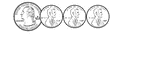### 28 Cents

Groups of change with totals from 1 to 100 cents using the least amount of coins.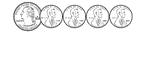### 29 Cents

Groups of change with totals from 1 to 100 cents using the least amount of coins.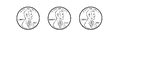### 3 Cents

Groups of change with totals from 1 to 100 cents using the least amount of coins.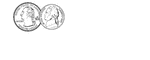### 30 Cents

Groups of change with totals from 1 to 100 cents using the least amount of coins.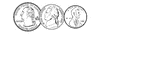### 31 Cents

Groups of change with totals from 1 to 100 cents using the least amount of coins.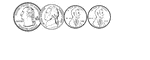### 32 Cents

Groups of change with totals from 1 to 100 cents using the least amount of coins.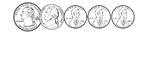### 33 Cents

Groups of change with totals from 1 to 100 cents using the least amount of coins.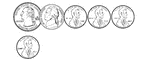### 34 Cents

Groups of change with totals from 1 to 100 cents using the least amount of coins.### 35 Cents

Groups of change with totals from 1 to 100 cents using the least amount of coins.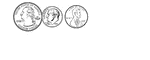### 36 Cents

Groups of change with totals from 1 to 100 cents using the least amount of coins.### 37 Cents

Groups of change with totals from 1 to 100 cents using the least amount of coins.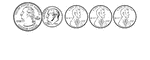### 38 Cents

Groups of change with totals from 1 to 100 cents using the least amount of coins.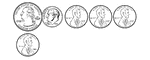### 39 Cents

Groups of change with totals from 1 to 100 cents using the least amount of coins.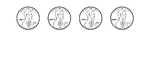### 4 Cents

Groups of change with totals from 1 to 100 cents using the least amount of coins.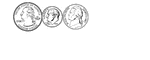### 40 Cents

Groups of change with totals from 1 to 100 cents using the least amount of coins.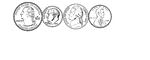### 41 Cents

Groups of change with totals from 1 to 100 cents using the least amount of coins.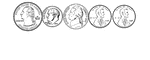### 42 Cents

Groups of change with totals from 1 to 100 cents using the least amount of coins.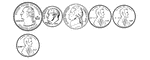### 43 Cents

Groups of change with totals from 1 to 100 cents using the least amount of coins.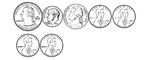### 44 Cents

Groups of change with totals from 1 to 100 cents using the least amount of coins.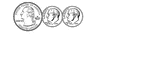### 45 Cents

Groups of change with totals from 1 to 100 cents using the least amount of coins.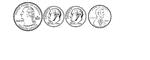### 46 Cents

Groups of change with totals from 1 to 100 cents using the least amount of coins.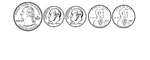### 47 Cents

Groups of change with totals from 1 to 100 cents using the least amount of coins.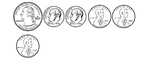### 48 Cents

Groups of change with totals from 1 to 100 cents using the least amount of coins.### 49 Cents

Groups of change with totals from 1 to 100 cents using the least amount of coins.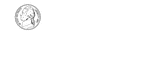### 5 Cents

Groups of change with totals from 1 to 100 cents using the least amount of coins.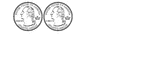### 50 Cents

Groups of change with totals from 1 to 100 cents using the least amount of coins.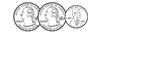### 51 Cents

Groups of change with totals from 1 to 100 cents using the least amount of coins.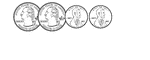### 52 Cents

Groups of change with totals from 1 to 100 cents using the least amount of coins.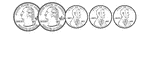### 53 Cents

Groups of change with totals from 1 to 100 cents using the least amount of coins.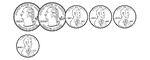### 54 Cents

Groups of change with totals from 1 to 100 cents using the least amount of coins.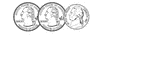### 55 Cents

Groups of change with totals from 1 to 100 cents using the least amount of coins.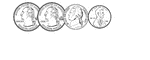### 56 Cents

Groups of change with totals from 1 to 100 cents using the least amount of coins.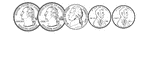### 57 Cents

Groups of change with totals from 1 to 100 cents using the least amount of coins.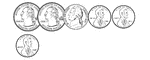### 58 Cents

Groups of change with totals from 1 to 100 cents using the least amount of coins.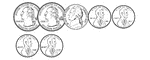### 59 Cents

Groups of change with totals from 1 to 100 cents using the least amount of coins.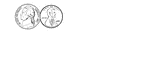### 6 Cents

Groups of change with totals from 1 to 100 cents using the least amount of coins.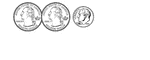### 60 Cents

Groups of change with totals from 1 to 100 cents using the least amount of coins.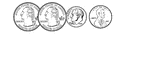### 61 Cents

Groups of change with totals from 1 to 100 cents using the least amount of coins.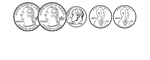### 62 Cents

Groups of change with totals from 1 to 100 cents using the least amount of coins.